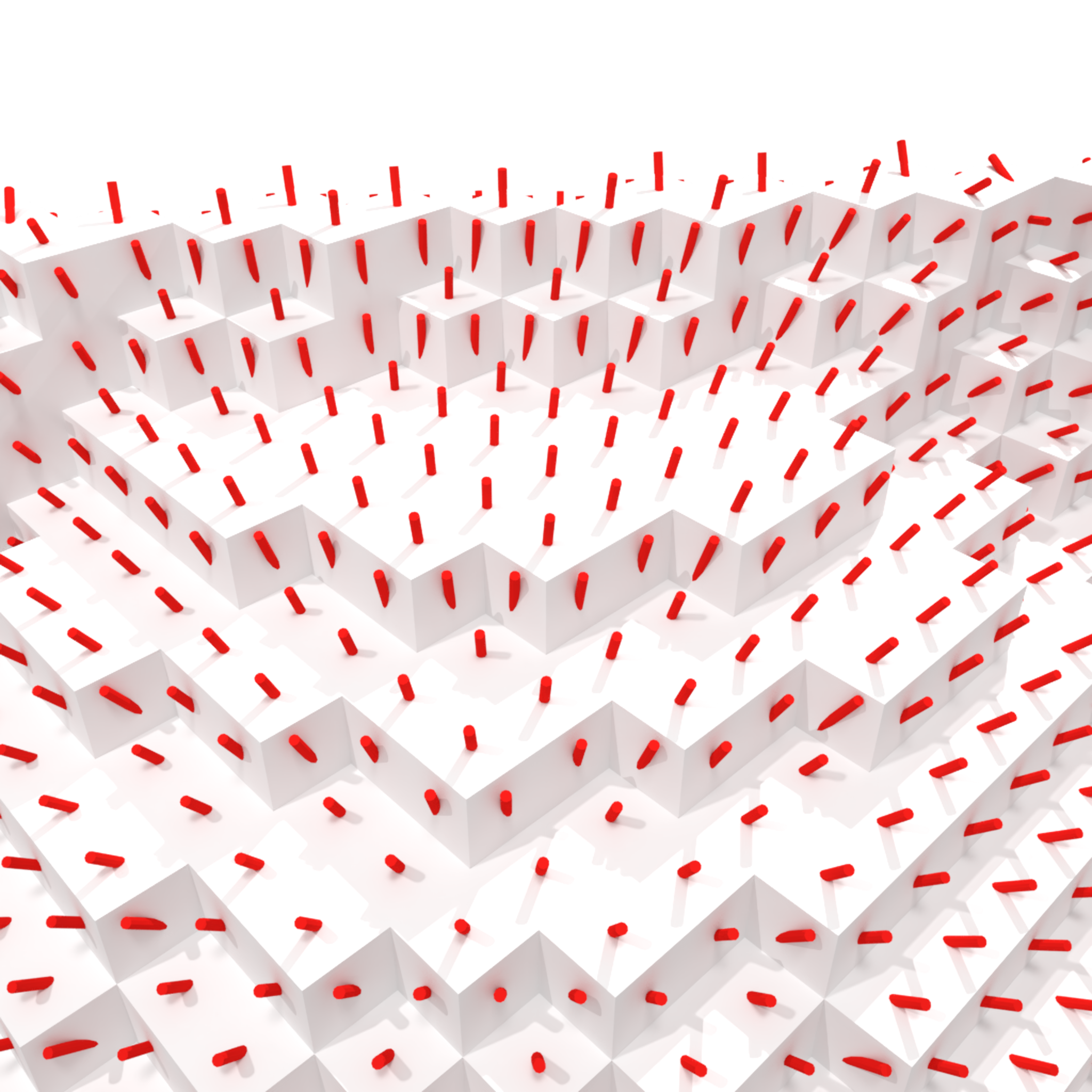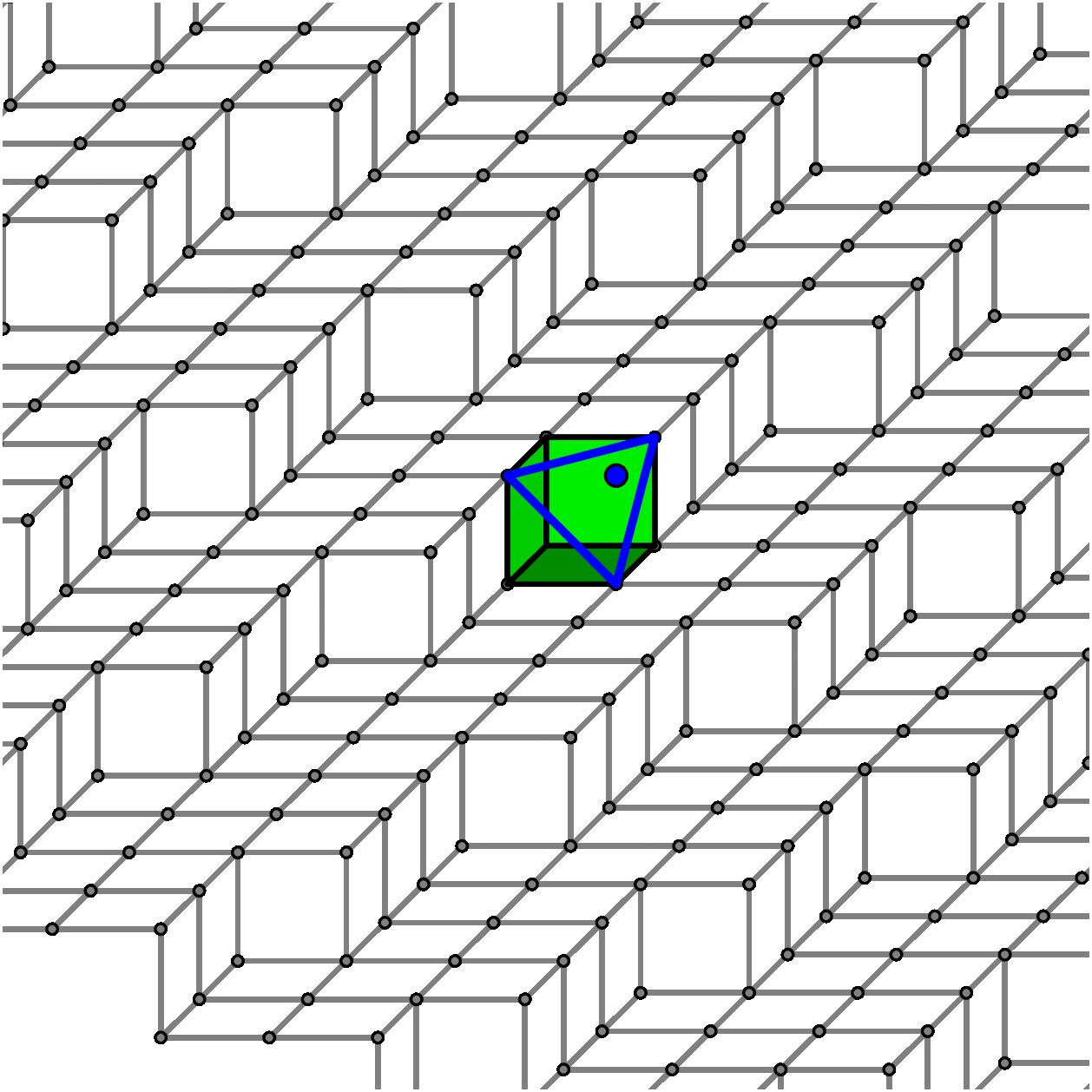Thesis of Jui-Ting Lu

Subject:
Parameter-free analysis of digital surfaces

Summary:

3D volumes come from the segmentation of magnetic resonance, X-ray tomographic or micro-tomographic images. They are also generated in scientific modelling and by voxel editors. We are interested in the geometry of volume boundaries, called digital surfaces (Fig. 1).1Figure 1: “ice-air” interface in a micro-tomographic image of snow.

Keeping the digital nature of the data is an advantage to use efficient spatial data structures such as voxel octree, to perform constructive solid geometry operations, to do integer-only and exact computations, etc. A drawback is its poor geometry, because at any resolution a digital surface is only made up of quadrangular surface element whose normal vector is parallel to one axis. Many tasks in computer graphics, vision and 3D image analysis require a richer geometry: rendering, surface deformation for simulation or tracking, precise geometric measurements, etc. To perform relevant geometric tasks and to benefit from the above-mentioned advantages in the same time, we need to enhance the geometry of digital surfaces by estimating extra data for each surfel, like a normal vector (Fig. 2). In order to estimate a relevant normal vector, the surface geometry within a patch around each surface element should be gathered. There are numerous methods, but almost all of them require at least one parameter that controls the size of the patch, which does not always reveal the local geometry.1Figure 2: Normal estimates onto a digital surface.

Objectives

We would like to work with a surface patch of adaptive size, which will be typically a piece of digital plane that locally fits the digital surface. In order to fulfill this objective, the main challenge is not really to recognize a piece of digital plane, but more to find which surface patch should be given as input to recognition algorithms. One alternative is to use a plane-probingalgorithm that decides on-the-fly which data points should be taken into account to make growing a piece of digital plane tangent to the surface by construction (see Fig. 3 and [LPR19LPR17LPR16bLPR16a]).Figure 3: Piece of digital plane onto a digital surface and its normal vector, equal to the normal vector of the computed triangular facet.

• A first objective is to derive, from plane-probing algorithms, efficient, accurate and parameter-free estimators of local and first-order geometric quantities: normal vector (and surfel area as a by-product), distance to boundary, voxel coverage.

• A second objective is to study the multigrid convergence of these estimators. Indeed, most of the time, when we are working with a digital surface, we are interested in the geometry of a continuous shape whose digitization is the input 3D volume. We expect that a given geometric quantity, such as a normal vector, computed at a point of a digital surface is close to the one of the underlying continuous shape at a close enough point. An estimator is multigrid-convergent if its accuracy depends on the resolution: the higher the resolution, the more accurate the estimator.

• Finally, plane-probing algorithms provide normal vector and position information, which may be useful for many applications in graphics such as polyhedral approximation, surface fairing or digital surface visualization. A third objective is to investigate at least one of these applications.